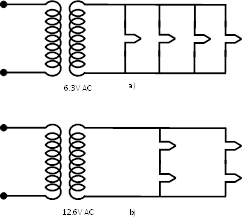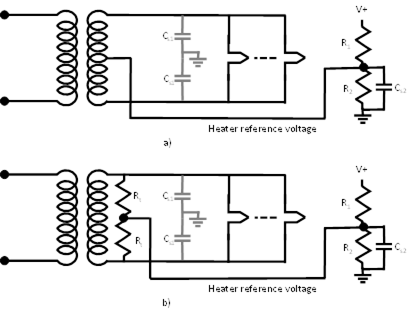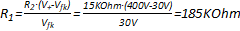The thermionic effect takes place when the cathode is heated at a very high temperature so that electrons start leaving the cathode surface. If the anode voltage is higher than the cathode, electrons emitted by the cathode are attracted by the anode, and current starts flowing from the anode to the cathode.

There are two methods to heat the cathode: the cathode can be directly heated or indirectly heated. In vacuum tubes with directly heated cathode, the heating filament also plays the role of the cathode, and electrons are emitted by the filament itself. In this case, DC voltage has to be used to heat the filament, in order not to introduce hum in the amplified signals. In vacuum tubes with indirectly heated cathodes, the filament and the cathode are two distinct components. The cathode is a metal cylinder, or some other shape, which surrounds the filament. The filament, in this case, is electrically isolated from the cathode. This allows AC voltage to be used to heat the filament.

Many vacuum tubes, used in audio applications, have indirectly heated cathodes. Here we will discuss only this heating method and we will consider the use AC voltage to supply power to the filaments.

Many vacuum tubes require a filament voltage of 6.3V, which can be obtained by connecting the filament to a step-down transformer, as shown in Figure 43 a). Some vacuum tubes, as for instance 12AX7 vacuum tubes, are internally composed of two different vacuum tubes. In this case, each internal filament be can be either powered with 6.3V or can be connected in series and powered with a voltage of 12.6V, as shown in Figure 43 b).

When choosing the power supply transformer, in addition to the output voltage, the current rating should be considered as well. Vacuum tubes datasheets generally report the current absorbed by the heaters.

 Example 23: Choosing heaters transformers An EL34 vacuum tube requires 6.3V at 1.5A. If we need to provide electric power to the heaters of a stereo push-pull amplifier, composed of four EL34, with filaments connected in parallel, we need a step-down transformer of 6.3V, rated at least for 6A. 12AX7 tubes contains two vacuum tubes each requiring 6.3V at 0.15A. Suppose we use two of them (thus, four vacuum tubes) for both the input and phase splitter stages. In this case, we have two options. We can power all of them in parallel, as in Figure 43 a), with a transformer of 6.3V rated for at least 0.6A. Alternatively, we connect the two internal heaters of each 12AX7 in series and then connect the two series in parallel, as in Figure 43 b), with a transformer of 12.6V rated for at least 0.3A.Figure 43: Heater’s power supply.Many vacuum tubes heaters requires 6.3V. All vacuum tubes heaters can be simply connected in parallel to a 6.3V transformer as show in a). Some vacuum tubes internally contain two distinct vacuum tubes, whose filament can be individually powered at 6.3V, or can be powered in series at 12.6V, as show in b).

### 5.2.1    Improved circuit: common and elevated voltage reference

Using the schemas given in Figure 43, the heater filaments are isolated from the other amplifier components. This implies that there is no common voltage reference and the filament voltage is floating, with respect to the voltage of the other amplifier components. The voltage difference between filament and cathode can assume uncontrolled large values. This can introduce hum in the audio signal, given to current leakage from the filament to the cathode. More importantly, an arc can occur between the filament and the cathode, which would damage the vacuum tubes.

Vacuum tubes datasheets generally report the maximum allowed positive and negative voltage between filament and cathode. This limit must be observed, to guarantee a safe operation of the vacuum tube. For instance, the Philips EL34 datasheet specify 100V as maximum filament to cathode voltage.

Generally, the voltage reference of the heater circuit is set higher than the cathode voltage, to reduce induced hum by eliminating current leakage between the filament and the cathode. In addition, in some configurations, cathode voltage is much higher that ground. Consider for instance a concertina phase splitter, where cathode voltage can be around 100V. In these cases, filament voltage must be elevated to be closer to the cathode voltage, and within the limits reported in the datasheets of the vacuum tubes.

Voltage reference can be set with a circuit like the one in Figure 44 a). A voltage divider composed of resistors R1 and R2, from high-tension V+ and ground, produces the needed elevated voltage reference. The voltage reference can be given to the filament power supply circuit using the centre tap of a centre tapped transformer. Note that, there is no current flowing from the filament power supply circuit to the voltage divider. The connection, from the voltage divider and the centre tap, just sets a common voltage reference. A smoothing capacitor Cs2 is generally used in the voltage divider, to attenuate voltage ripple and other interferences. Large capacitance values, as for instance 1μF, or even 10μF, are generally used for the smoothing capacitor Cs2.Figure 44: Heater’s power supply elevated voltage referenceVoltage reference between filament and cathode can be set using a voltage divider, from V+ to ground, and feeding it through a centre tapped transformer as shown in a). In case the transformer does not have a centre tap, an artificial centre tap can be obtained connecting two appropriate resistors Rt between the two transformer ends, and feeding the voltage reference through them, as shown in b). It is generally convenient to decouple the lower resistor of the voltage divider with a large capacitor Cs2. Sometimes, it is also convenient to use the two capacitors Cs1 to short to ground current peaks due, for instance, to solid-state rectifier switching interferences, which can transfer electromagnetic noise to the cathode.

Generally, R1 and R2 have large values, in order not to have too much current in the voltage divider. However, vacuum tube datasheets generally specify also the maximum filament to cathode resistance that can be tolerated, in addition to the above-mentioned filament to cathode voltage. This poses a limit to the maximum allowed value of R2. For instance, the Philips EL34 datasheet specify 20K Ohms as maximum filament to cathode resistance.

 Example 24: Heater voltage elevation Suppose, we have an EL34 configured in fixed bias, so that its cathode is connected to ground. Suppose that the anode voltage V+ is 400V. We already said that Philips EL34 datasheets specify that maximum filament to cathode voltage is 100V and that maximum filament to cathode resistance is 20K Ohms. Accordingly, suppose that we want to set the filament to cathode voltage Vfk to 30V and that we want to have a filament to cathode resistance, corresponding to R2, equal to 15K Ohms. Using the voltage divider equation, we have that. The closest standard is R1=180K Ohms. The current dissipated by the voltage divider is 400V/(15K Ohms+180K Ohms)=2.05mA. The power dissipated by the two resistors R1 and R2 is respectively (2.05mA)2∙180K Ohms=0.76 W and (2.05mA)2∙15K Ohms =0.06 W.

### 5.2.2    Artificial transformer centre tap

Sometimes, heater transformers do not have a centre tap. In these cases, an artificial centre tap can be easily created by connecting two resistors Rt to the two transformer ends, as shown in Figure 44 b). The voltage reference, produced by the voltage divider, can be fed through these two resistors, as shown in the figure. Note that this resistance should be added to the heater voltage elevation circuit resistance and should be taken into consideration when checking that the maximum cathode to filament resistance is not exceeded.

Generally, the two resistors Rt have a resistance around 100 or 220 Ohms. As we said previously, there is no current flowing from the voltage divider to the filament power supply circuit. However, current flows across the two resistors Rt, from one transformer end to the other. Suppose we use two 100 Ohms resistors. The current is 6.3V/200 Ohms=31.5mA and the dissipated power is (31.5mA)2∙200 Ohms=0.2W.

Sometimes, a potentiometer is used in place of the two resistors Rt and the reference voltage produced by the voltage divider is fed through the potentiometer wiper terminal. The potentiometer allows varying the values of the resistance between the artificial centre tap and the two transformer ends, to find the position where the hum, possibly introduced by the filament power supply circuit, is minimized.

### 5.2.3    Reducing electromagnetic interferences from other transformer windings

In many cases, instead of using several power supply transformers, one single transformer is used, which contains several independent windings for the various amplifier components. One of these is the heater power supply winding. In these cases, there could be interferences between one section and the others. For instance, consider that very short and intense current impulses go through the transformer windings connected to solid state rectifiers, when using large values of reservoir capacitors. Impulses have 50Hz or 100Hz frequency, depending on the type of rectifier. They are very short and intense, and also contain very high frequency components that produce electromagnetic interferences that may travel across the various sections of the transformer. When these interferences reach the filament winding, they can also interfere with the cathode and can be heard as a buzzing noise. The interferences can be significantly reduced, by shorting them to ground, using low value capacitors connected from the two ends, of the heaters’ transformer, to ground. This is depicted by the two greyed Cs1capacitors in Figure 44 a). Small capacitance values, for instance 6.8 nF, can be used to accomplish to this task.